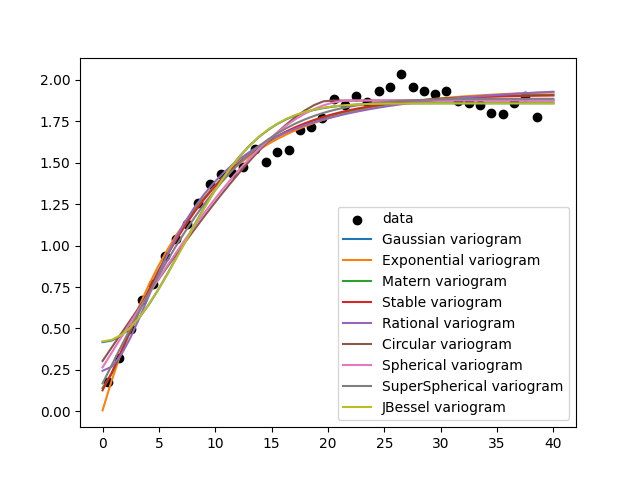# Finding the best fitting variogram model

```import numpy as np
from matplotlib import pyplot as plt

import gstools as gs
```

Generate a synthetic field with an exponential model.

```x = np.random.RandomState(19970221).rand(1000) * 100.0
y = np.random.RandomState(20011012).rand(1000) * 100.0
model = gs.Exponential(dim=2, var=2, len_scale=8)
srf = gs.SRF(model, mean=0, seed=19970221)
field = srf((x, y))
```

Estimate the variogram of the field with 40 bins and plot the result.

```bins = np.arange(40)
bin_center, gamma = gs.vario_estimate((x, y), field, bins)
```

Define a set of models to test.

```models = {
"Gaussian": gs.Gaussian,
"Exponential": gs.Exponential,
"Matern": gs.Matern,
"Stable": gs.Stable,
"Rational": gs.Rational,
"Circular": gs.Circular,
"Spherical": gs.Spherical,
"SuperSpherical": gs.SuperSpherical,
"JBessel": gs.JBessel,
}
scores = {}
```

Iterate over all models, fit their variogram and calculate the r2 score.

```# plot the estimated variogram
plt.scatter(bin_center, gamma, color="k", label="data")
ax = plt.gca()

# fit all models to the estimated variogram
for model in models:
fit_model = models[model](dim=2)
para, pcov, r2 = fit_model.fit_variogram(bin_center, gamma, return_r2=True)
fit_model.plot(x_max=40, ax=ax)
scores[model] = r2
```Create a ranking based on the score and determine the best models

```ranking = sorted(scores.items(), key=lambda item: item, reverse=True)
print("RANKING by Pseudo-r2 score")
for i, (model, score) in enumerate(ranking, 1):
print(f"{i:>6}. {model:>15}: {score:.5}")

plt.show()
```
```RANKING by Pseudo-r2 score
1.          Stable: 0.98218
2.          Matern: 0.98176
3.  SuperSpherical: 0.98141
4.     Exponential: 0.98041
5.        Rational: 0.97711
6.       Spherical: 0.97334
7.        Circular: 0.96725
8.        Gaussian: 0.95928
9.         JBessel: 0.95831
```

Total running time of the script: ( 0 minutes 1.102 seconds)

Gallery generated by Sphinx-Gallery